Optics
Volume 4, Issue 3-1, June 2015, Pages: 14-17

Measurement of Distortion Using the Moire Interferometry

K. Wenzel, Gy. Abraham, P. Tamas, A. Urbin

Department of Mechatronics, Optics and Information Engineering, Faculty of Mechanical Engineering, Budapest University of Technology and Economics, Budapest, Hungary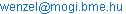(K. Wenzel)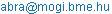(G. Ábrahám)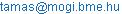(P. Tamás)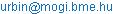(Á. Urbin)

K. Wenzel, Gy. Ábrahám, P. Tamás, Á. Urbin. Measurement of Distortion Using the MoiréInterferometry. Optics. Special Issue: Optical Techniques for Deformation, Structure and Shape Evaluation. Vol. 4, No. 3-1, 2015, pp. 14-17. doi: 10.11648/j.optics.s.2015040301.14

Abstract: Image forming lens systemsdo not provide perfect images. Lens abberations can be detected with complex optical ray-tracing. A single aberration in lenses, the distortion, can be detected at the image plane with optical mapping. Therefore moiré interferometry can be adapted to detect and measure distortion. This method is adaptable by serial testing of the universal measuring cameras, projection cameras, photogrammetrical cameras, etc. The distortion of the whole image plane can be represented on one moiré image. This can be useful if the distortion is not rotationally symmetrical for example because of errors of assembly. The distortion of 1 µm regarding the focal plane can be measured by using the moiré method presented in this paper.

Keywords: Lens Aberrations, Moire Method, Distortion

1. Introduction

Image forming optical lens systems do not provide perfect images. The image aberrations were categorised and defined with third order sine functions by L. Seidel in 1856 . These image defects are defined as third order aberrations. The monochromatic aberrations (spherical aberration, coma, astigmatism, curvature of field anddistortion) exist for any specified color and refractive index. However the chromatic aberrations (longitudinal chromatic aberration and lateral chromatic aberration) have different magnitude at different wavelengths.

As distortion is independent of the wavelength, and moreover it appears in the image plane, it can be detected and measured with the moiréinterferometry.

Experimental detection of distortion is realized generally by applying split-scales of known distances. The split-scale will be placed in the object plane of the objective under test and projected in the image plane. The radial displaces in the picture of the split-scale are measured either directly, visually by applying a reference scale and suitable microscope, or by using a photographic evaluating process, eventually also by applying an optoelectronic measuring system .

In the course of this process the moiré interferometry could be usefully adapted.

A transmission grating produced microlithographically is placed in the object plane of the objective under test. The grating is illuminated incoherently and projected by the unknown objective in the image plane. A second transmission grating produced also microlitographically is placed exactly in the image plane, and there the moiré effect appears.

The moiré fringes indicate the distortion. They are perceptible either directly, visually by using a camera or by applying a CCD detector and a computer to evaluate the measuring data.

This method is adaptable by serial testing of the universal measuring cameras, projection cameras, photogrammetrical cameras, etc. One μm distortion is perceptible regarding the focal plane of the objective under test.

2. The Moiré-Effect

The moiré phenomenon and some metrological applications of it are reviewed e.g. in [5,6,7,8]. The moiré pattern of two parallel line transmission gratings is shown in Fig. 1.

The spatial frequencies of the gratings are ν1 and ν2. They are dissimilar. Their patterns are the basic patterns of the picture.But there is a new pattern in the picture: the moiré pattern. Its spatial frequency is νm.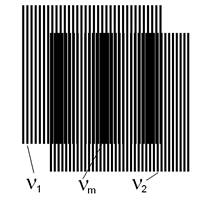Fig. 1. Moiré pattern of two transmission gratings with parallel lines,ν1 and ν2 are the spatial frequencies of the two transmission gratings;νm is the spatial frequency of the moiré pattern.

Let transmission coefficients of the gratings be τ1 and τ2. The values of τ1 and τ2 depend on x:

τ1=0 if cos2π ν1x≤0

τ1=1 if cos2π ν1x>0

and

τ2=0 if cos2πν2x≤0

τ2=1 if cos2πν2x>0             (1)

where x≥1 isinteger.

Common transmission coefficient of the two transmission gratings is τ12:

τ12 = τ1τ2                   (2)

From equation (1) and equation (2)

τ12=0 if cos2π ν1xcos2π ν2x=0

or τ12=0,

τ12=1 otherwise.                (3)

Using the identity

cosα cosβ = ½ cos(α + β) + ½ cos (α - β)

τ12=0 if cos2πx(ν12)+cos2πx(ν12)=0

or τ12=0,

τ12=1 otherwise.                (4)

In equation (4) the sum of frequencies is the sum of spatial frequencies of the basic patterns and the difference of frequencies is the spatial frequency of moiré fringes.

Since (ν1)>>(ν12) and (ν2)>>(ν12), the basic patterns are filterable and the moiré pattern is clearly visible and detectable.

The condition of appearance of n complete moiré fringes comes from equation (4):

x(ν12)=n;                    (5)

where n≥1 is integer.

3. The Distortion

Even if an optical system were designed so that the first four Seidel aberration were zero, it could still be affected by the fifth aberration: the distortion. To be free of distortion a system must have uniform lateral magnification over its entire field. A pinhole-camera is ideal in this respect,because it shows no distortion; all straight lines connecting each pair of conjugate points in the object and image planes pass through the pin hole .Fig. 2. A pinhole-camera shows no distortion.

Constant magnification for a pinhole camera as well as for a lens implies, as may be seen from Fig. 2, that tanΦ’/tanΦ=const.

The common forms of image distortion produced by lenses are illustrated in Fig. 3. The diagram (a) represents the undistorted image of an object consisting of a rectangular wire mesh. The diagram (b) shows "barrel distortion", which arises when the magnification decreases toward the edge of the field. The diagram (c) represents "pincushion distortion", corresponding to a greater magnification at the borders.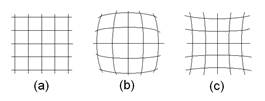Fig. 3. A pinhole-camera shows no distortion. Images of a rectangular object screen shown with (a) no distortion; (b) barrel distortion; (c) pincushion distortion.

Definition of distortion is reviewed e.g. in .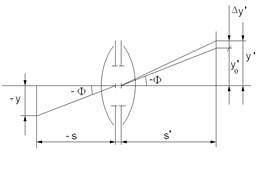Fig. 4. Fundamentals of distortion.

Using the signs of Fig. 4,the distortion is Δy’.

On the basis of Fig. 4

y0’ = β y,                  (6)

y’ = y0’ + Δy’                 (7)

where y is object size, y0’ is correct size of image, y is image size if distorsion is present, Δy’ is distortion, β is magnification, s is object distance and s’ is image distance.

In case, if the transmission gratings are both in the object plane and the picture plane, the moiré fringes appear in the picture plane. The number of moiré fringes is proportional to the distortion . Namely in equation (5) letx=y’be thesize of distorted image, ν1=v0be thespatial frequency of the correct image, ν=ν’be the spatial frequencyof the distorted image, and N=y’ν’=y0’ν0’be thenumber of the basic line in the object plane or in the image plane.

From equation (5) and equation (7) we have

n = Δy’v0                (8)

Consequently the number of moiré fringesn is proportional tothe distortion Δy’really

4. Measurement of Distortion Using the Moiré Interferometry

The optical scheme of the measuring system is shown in Fig. 5.

Two moiré pictureswill be made during the measuring. One will be made in the 1:1 image plane and the other inthe 2:1 image plane .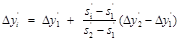(9)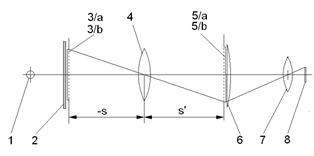Fig. 5. Optical scheme of the measuring system, 1 is incoherent light source; 2 is mat glass plate; 3/a and 3/b are transmission gratings (object gratings); 4 is objective under test; 5/a and 5/b are transmission gratings (analyser gratings); 6 is field lens; 7 is objective for observation; 8 is CCD line element.

The steps of the measuring method are:

1.   rough adjustment in the 1:1 image plane using the 3/a and 5/a transmission gratings (see Fig. 5.); the moiré lines will appear,

2.   fine adjustment, minimization of the moiré lines,

3.   registration of the moiré lines,

4.   rough adjustment in the 2:1 image plane using the 3/b and 5/b transmission gratings (see Fig. 5),

5.   fine adjustment, minimization of the moiré lines,

6.   registration of the moiré lines,

7.   calculation of distortion in the focal plane or in any other plane using equation (8).

5. Calculation of Distortion in Any Image Plane

Distortion is proportional to the image distance. Fig. 6 shows the situation in case of the general centered (rotationally symmetrical) optical system.

On the basis of Fig. 6

The calculation is given by J. Menz for both a rotationally symmetrical optical system and an asymmetrical optical system.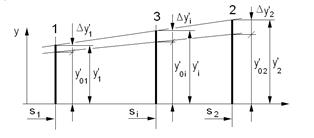Fig. 6. Distortion is proportional to the image distance, 1is 1:1 image plane;2is 2:1 image plane; 3is any image plane; s1, s2 and s3 are the appropriate image distances.

6. Distortion Measurement Sensitivity

The measuring parameters depend on the transmission gratings 3/a, 3/b, 5/a, 5/b. Microlitographically produced gratings  can be used in the form shown in Fig. 7. Used the gratings shown in Fig. 7, one moiré fringe indicates 1 µm distortion regarding the focal plane.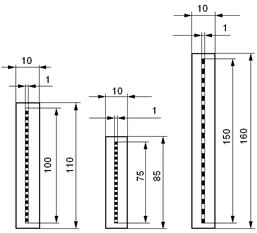Fig. 7. Transmission gratings applied for the measuring, the left are gratings 3/a and 5/a, ν=500 lp/mm; the middle is grating 3/b, ν=666.6 lp/mm; and the right is grating 5/b, ν=333.3 lp/mm.

The distortion of the whole image plane can be represented on one moiré image if the gratings 3/a and 3/b, that cover the whole object plane, are set into the object plane, the gratings 5/a and 5/b, that also cover the whole object plane, are set into the image plane, besides as a detector a planar CCD detector is applied instead of a linear one. This can be useful if the distortion is not rotationally symmetrical for example because of errors of assembly .

7. Results and Discussion

A single error in lenses, the distortion, can be detected at the image plane with optical mapping. Therefore moiré interferometry can be adapted to detect and measure distortion. This method is adaptable by serial testing of the universal measuring cameras, projection cameras, photogrammetrical cameras, etc. The distortion of the full display can be represented on one moiré image. This can be useful if the distortion is not rotationally symmetrical for example because of errors of assembly. The distortion of 1 µm regarding the focal plane can be measured by using the moiré method presented in this paper.

8. Conclusions

In this paper, the moiré inter ferometry was used to measure the distortion of image forming optical lens systems. Microlito graphically produced transmission gratings were placed in the object plane and in the image plane. The moire picture appears in the image plane. The moiré fringes indicate the distortion. They are perceptible either directly, visually by using a camera or by applying a CCD detector and a computer to evaluate the measuring data.

References

1. F.A.Jenkins andH.E.White,"Fundamentals of optics"4th ed.,McGraw-Hill,1976, pp 171-173.
2. U.Untergutsch,"Fotoelektrische Verzeichnungsmeßung an MSP-4 Objektiven", Feingerätetechnik 29, 1980 H. 4.
3. L.A.Sayce,"Gratings in metrology", Journal of Physics E: Scientific Instruments,Vol. 5. 1972, pp. 193-198.
4. J.Menz,"Verzeichnung optischer Systeme und ihre rechnerische Berücksichtigung bei Meßaufgaben", Feingerätetechnik 29, 1980.
5. K.J.Gasvik,"Optical metrology",J.Wiley & Sons, 1987.
6. O.Kafri and I.Glatt,"The physics of moiré metrology", John Wiley & Sons Inc.
7. P.S.Theocaris,"Moiré fringe in strain analysis", Pergamon Press, 1969.
8. K.Patorski and M.Kujawinska,"Handbook of the moiré fringe technique", Elsevier,Amsterdam, 1993.
9. K.Wenzel and N.Tveritinova,"Shape identification using moire topography", Periodica Polytechnica, Vol.43. No.1. 1999, pp. 47-54.
10. A.Lohmann,"Das Moiré-Gitter als vielseitiges Testobjekt: Photoelektrische Aberrationsmessung", Optica Acta, Vol. 6. 1959. pp. 37-41.
11. K.Wenzel, A.Farkas, Á.Antal and Z.Musch,"Two exposure projection moiré method for measurement of forms, displacement and deformations", Interferometry’89, Warshaw, May, 1989.
12. M.D.Ibrahim,"Formation of unsymmetric moiré fringes and their use in moire topography", Optics and Laser in Engineering, Vol. 3. 1982, pp. 65-72.

 Contents 1. 2. 3. 4. 5. 6. 7. 8.
Article ToolsAbstractPDF(200K)